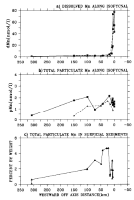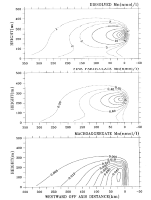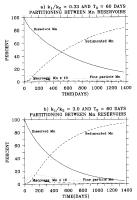U.S. Dept. of Commerce / NOAA / OAR / PMEL / Publications

## A Model for the Deposition of Hydrothermal Manganese Near Ridge Crests

### Gallery of FiguresFigure 1. (a) Dissolved and (b) total particulate Mn as a function of distance normal to the Cleft segment of the JDFR ridge as measured (Table 1) in 1985 (solid curve) and 1986 (dashed curve). (c) Total particulate Mn as weight percent in surficial sediments (Table 2).Figure 2. Diagram of the Mn reservoirs and exchange pathways incorporated in the scavenging model. The physical processes of advection and diffusion are not represented.Figure 3. Model results under parameter conditions of Table 3 showing off-axis transects of (a) dissolved manganese (dMn = T) and (b) total particulate manganese (pMn = T+ T) at heights of (solid) 240 m and (dashed) 120 m. (c) Model pMn flux (mg/cm/kyr) to the seafloor (solid curve) and measured weight percent of Mn in surficial sediments (squares, Table 2).Figure 4. Off-axis concentration distributions (T, T, T) of the three water column phases of hydrothermal Mn (nmol/L).Figure 5. (a) Distributions of model Mn flux to sediments (curves) for varying off-axis advection velocities when T= 60 days. Superimposed on model distributions are the measured weight percent of Mn in surficial sediments (squares) from Table 2. Flux distributions have been individually rescaled so that maximum has the same ordinate value as the data maximum; flux scales are consequently not shown. The degree of misfit between each distribution and the measured weight percents was calculated as the sum of squared errors, as described in the text. (b) The resulting sum-of-squared-error surfaces for a range of u and Tvalues when k/kequals 0.33 and (c) when k/kequals 3.Figure 6. Percent of initial discharge in each of four Mn reservoirs as a function of time. Parameter settings are as in Table 3, but with (a) k/k= 0.33 and (b) k/k= 3.